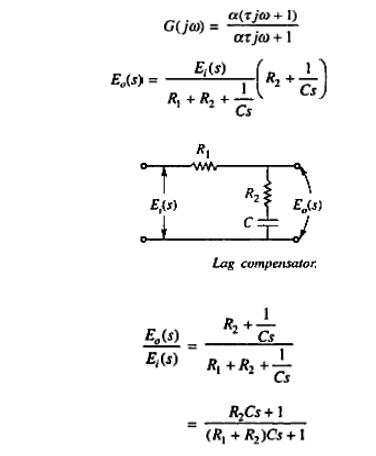Home | | Control System Engineering | Types of Compensation

# Types of Compensation

Series Compensation or Cascade Compensation, Feedback compensation or Parallel compensation, Series-feedback compensation, Feed forward compensation, Lead Compensator, Lag Compensator, Lag-Lead Compensator

TYPES OF COMPENSATION

## Series Compensation or Cascade Compensation

This is the most commonly used system where the controller is placed in series with the controlled process.

Figure shows the series compensation### Feedback compensation or Parallel compensation

This is the system where the controller is placed in the sensor feedback path as shown in fig.## State Feedback Compensation

This is a system which generates the control signal by feeding back the state variables through constant real gains. The scheme is termed state feedback. It is shown in Fig.The compensation schemes shown in Figs above have one degree of freedom, since there is only one controller in each system. The demerit with one degree of freedom controllers is that the performance criteria that can be realized are limited.

That is why there are compensation schemes which have two degree freedoms, such as:

(a)Series-feedback compensation

(b) Feed forward compensation

## Series-Feedback Compensation

Series-feedback compensation is the scheme for which a series controller and a feedback controller are used. Figure 9.6 shows the series-feedback compensation scheme.## Feed forward Compensation

The feed forward controller is placed in series with the closed-loop system which has a controller in the forward path Orig. 9.71. In Fig. 9.8, Feed forward the is placed in parallel with the controller in the forward path. The commonly used controllers in the above-mentioned compensation schemes are now described in the section below.It has a zero and a pole with zero closer to the origin. The general form of the transfer function of the load compensator is## Lag Compensator

It has a zero and a pole with the zero situated on the left of the pole on the negative real axis. The general form of the transfer function of the lag compensator isTherefore, the frequency response of the above transfer function will beThe lag-lead compensator is the combination of a lag compensator and a lead compensator. The lag-section is provided with one real pole and one real zero, the pole being to the right of zero, whereas the lead section has one real pole and one real came with the zero being to the right of the pole.

The transfer function of the lag-lead compensator will beStudy Material, Lecturing Notes, Assignment, Reference, Wiki description explanation, brief detail
Control Systems : Frequency Response Analysis : Types of Compensation |